# Decision tree

6 Sep 2011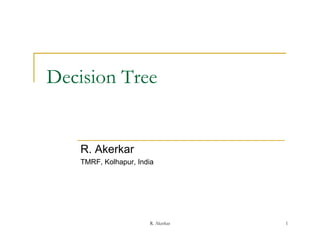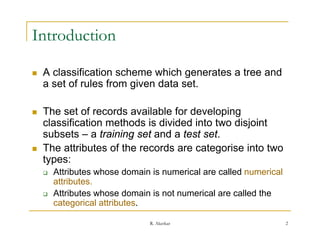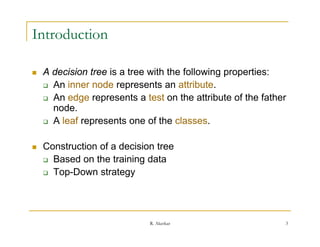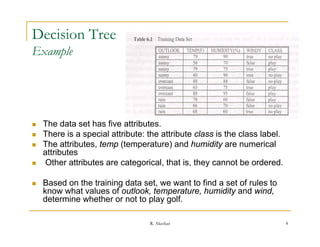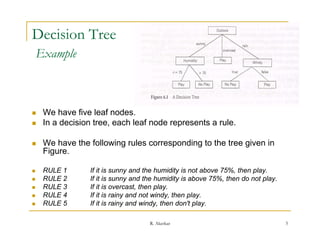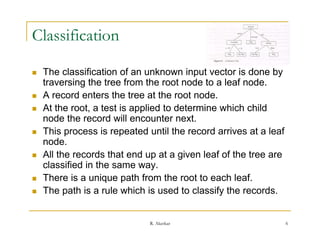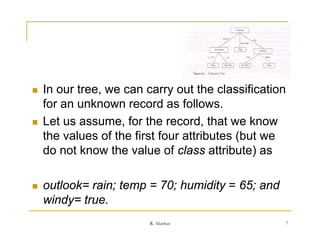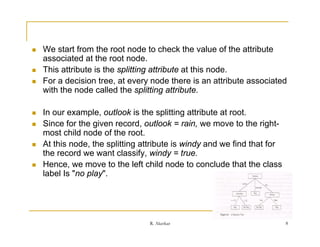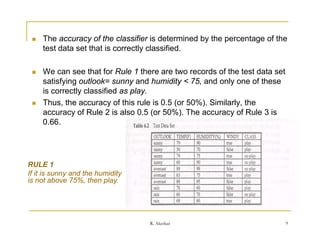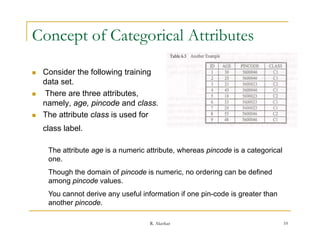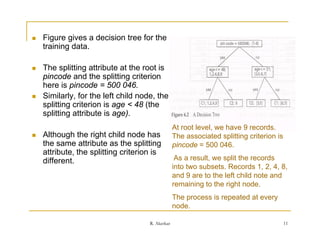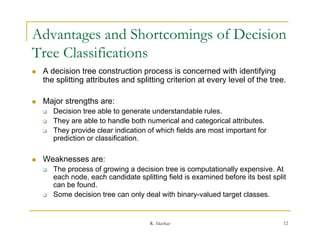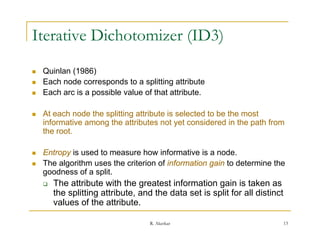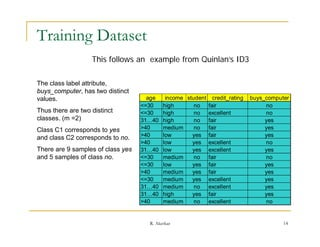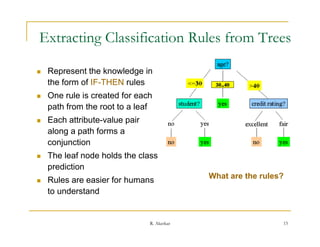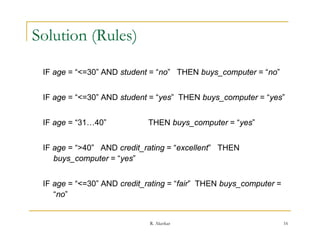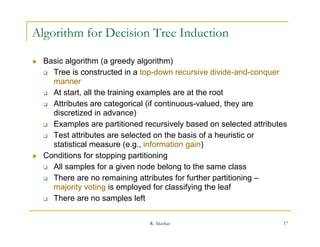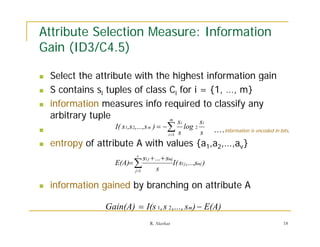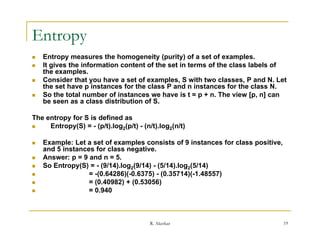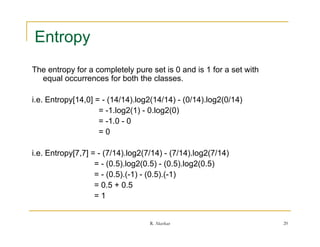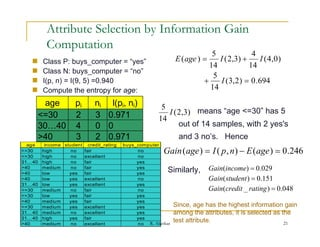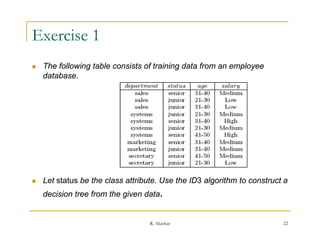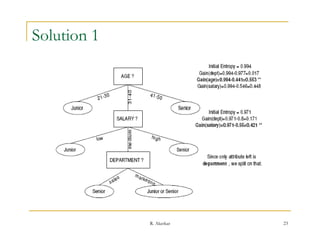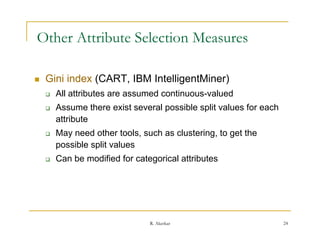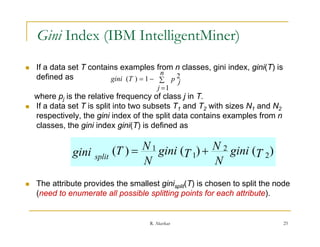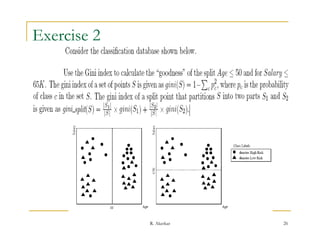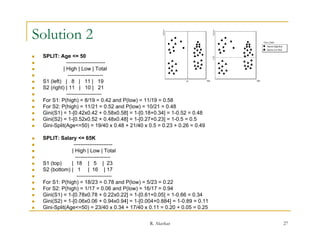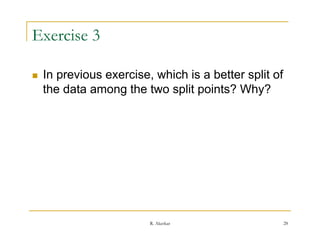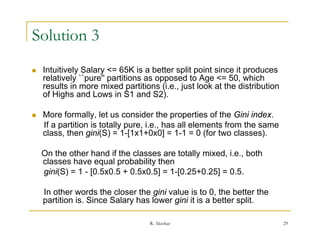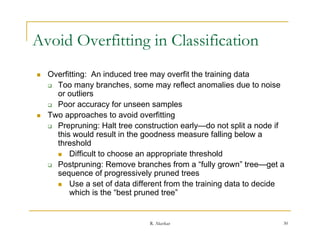1 sur 30

### Decision tree

• 1. Decision Tree R. Akerkar TMRF, Kolhapur, India R. Akerkar 1
• 2. Introduction  A classification scheme which generates a tree and g a set of rules from given data set.  The t f Th set of records available f d d il bl for developing l i classification methods is divided into two disjoint subsets – a training set and a test set. g  The attributes of the records are categorise into two types:  Attributes hose Attrib tes whose domain is n merical are called n merical numerical numerical attributes.  Attributes whose domain is not numerical are called the categorical attributes attributes. R. Akerkar 2
• 3. Introduction  A decision tree is a tree with the following p p g properties:  An inner node represents an attribute.  An edge represents a test on the attribute of the father node. node  A leaf represents one of the classes.  Construction of a decision tree  Based on the training data  Top Down strategy Top-Down R. Akerkar 3
• 4. Decision Tree Example  The data set has five attributes.  There is a special attribute: the attribute class is the class label.  The attributes, temp (temperature) and humidity are numerical attributes  Other attributes are categorical, that is, they cannot be ordered.  Based on the training data set, we want to find a set of rules to know what values of outlook, temperature, humidity and wind, determine whether or not to play golf. R. Akerkar 4
• 5. Decision Tree Example  We have five leaf nodes.  In a decision tree, each leaf node represents a rule.  We have the following rules corresponding to the tree given in Figure.  RULE 1 If it is sunny and the humidity is not above 75% then play 75%, play.  RULE 2 If it is sunny and the humidity is above 75%, then do not play.  RULE 3 If it is overcast, then play.  RULE 4 If it is rainy and not windy, then play.  RULE 5 If it is rainy and windy, then don't play. i i d i d th d 't l R. Akerkar 5
• 6. Classification  The classification of an unknown input vector is done by p y traversing the tree from the root node to a leaf node.  A record enters the tree at the root node.  At the root, a test is applied to determine which child root node the record will encounter next.  This process is repeated until the record arrives at a leaf node. d  All the records that end up at a given leaf of the tree are classified in the same way. y  There is a unique path from the root to each leaf.  The path is a rule which is used to classify the records. R. Akerkar 6
• 7. In our tree, we can carry out the classification for a u o an unknown record as follows. o eco d o o s  Let us assume, for the record, that we know the values of the first four attributes (but we do not know the value of class attribute) as  outlook= rain; temp = 70; humidity = 65; and windy true. windy= true R. Akerkar 7
• 8. We start from the root node to check the value of the attribute associated at the root node.  This attribute is the splitting attribute at this node.  For a decision tree, at every node there is an attribute associated with the node called the splitting attribute.  In our example, outlook is the splitting attribute at root.  Since for the given record, outlook = rain, we move to the right- most child node of the root.  At this node, the splitting attribute is windy and we find that for the record we want classify, windy = true.  Hence, we move to the left child node to conclude that the class label Is "no l " l b l I " play". R. Akerkar 8
• 9. The accuracy of the classifier is determined by the percentage of the test d t t t data set that is correctly classified. t th t i tl l ifi d  We can see that for Rule 1 there are two records of the test data set satisfying outlook= sunny and humidity < 75, and only one of these is correctly classified as play.  Thus, the accuracy of this rule is 0.5 (or 50%). Similarly, the accuracy of Rule 2 is also 0.5 (or 50%). The accuracy of Rule 3 is 0.66. RULE 1 If it is sunny and the humidity is not above 75%, then play. R. Akerkar 9
• 10. Concept of Categorical Attributes  Consider the following training data set.  There are three attributes, namely, age, pincode and class.  The attribute class is used for class label. The attribute age is a numeric attribute, whereas pincode is a categorical one. Though th d Th h the domain of pincode i numeric, no ordering can b d fi d i f i d is i d i be defined among pincode values. You cannot derive any useful information if one pin-code is greater than another pincode pincode. R. Akerkar 10
• 11. Figure gives a decision tree for the training data data.  The splitting attribute at the root is pincode and the splitting criterion here is pincode = 500 046.  Similarly, for the left child node, the splitting criterion is age < 48 (the p g g ( splitting attribute is age). At root level, we have 9 records.  Although the right child node has The associated splitting criterion is p g the same attribute as the splitting pincode = 500 046. attribute, the splitting criterion is different. As a result, we split the records into two subsets. Records 1, 2, 4, 8, and 9 are to the left child note and remaining to the right node. The process is repeated at every node. R. Akerkar 11
• 12. Advantages and Shortcomings of Decision Tree Classifications  A decision tree construction process is concerned with identifying the splitting attributes and splitting criterion at every l h li i ib d li i i i level of the tree. l f h  Major strengths are:  Decision tree able to generate understandable rules.  They are able to handle both numerical and categorical attributes.  They provide clear indication of which fields are most important for prediction or classification classification.  Weaknesses are:  The process of growing a decision tree is computationally expensive At expensive. each node, each candidate splitting field is examined before its best split can be found.  Some decision tree can only deal with binary-valued target classes. R. Akerkar 12
• 13. Iterative Dichotomizer (ID3)  Quinlan (1986)  Each node corresponds to a splitting attribute  Each arc is a possible value of that attribute.  At each node the splitting attribute is selected to be the most informative among the attributes not yet considered in the path from the root.  Entropy is used to measure how informative is a node.  The algorithm uses the criterion of information gain to determine the goodness of a split. d f lit  The attribute with the greatest information gain is taken as the splitting attribute, and the data set is split for all distinct values of the attribute attribute. R. Akerkar 13
• 14. Training Dataset This follows an example from Quinlan’s ID3 The class label attribute, buys_computer, has two distinct values. age income student credit_rating buys_computer <=30 high g no fair no Thus there are two distinct <=30 high no excellent no classes. (m =2) 31…40 high no fair yes Class C1 corresponds to yes >40 medium no fair yes and class C2 corresponds to no no. >40 low yes fair yes >40 low yes excellent no There are 9 samples of class yes 31…40 low yes excellent yes and 5 samples of class no. <=30 medium no fair no <=30 low yes fair yes >40 40 medium di yes f i fair yes <=30 medium yes excellent yes 31…40 medium no excellent yes 31…40 high yes fair yes >40 medium no excellent no R. Akerkar 14
• 15. Extracting Classification Rules from Trees g  Represent the knowledge in the form of IF-THEN rules  One rule is created for each path from the root to a leaf  Each attribute-value pair along a path forms a conjunction  The leaf node holds the class p prediction What are the rules?  Rules are easier for humans to understand R. Akerkar 15
• 16. Solution (Rules) IF age = “<=30” AND student = “no” THEN buys_computer = “no” IF age = “<=30” AND student = “yes” THEN buys_computer = “yes” IF age = “31…40” THEN buys_computer = “yes” IF age = “>40” AND credit_rating = “excellent” THEN buys_computer = “yes” IF age = “<=30” AND credit_rating = “fair” THEN buys_computer = “no” R. Akerkar 16
• 17. Algorithm for Decision Tree Induction  Basic algorithm (a greedy algorithm)  Tree is constructed in a top-down recursive divide-and-conquer manner  At start, all the training examples are at the root  Attributes are categorical ( continuous-valued, they are g (if y discretized in advance)  Examples are partitioned recursively based on selected attributes  Test attributes are selected on the basis of a heuristic or statistical measure (e.g., information gain)  Conditions for stopping partitioning  All samples for a g p given node belong to the same class g  There are no remaining attributes for further partitioning – majority voting is employed for classifying the leaf  There are no samples left p R. Akerkar 17
• 18. Attribute Selection Measure: Information Gain (ID3/C4.5) (ID3/C4 5)  Select the attribute with the highest information gain  S contains si tuples of class Ci for i = {1, …, m}  information measures info required to classify any q y y arbitrary tuple m si si I( s1,s 2,...,s m )    log 2  i 1 s s ….information is encoded in bits.  entropy of attribute A with values {a1,a2,…,av} f b h l { v s1 j  ... smj E(A)  I ( s1 j ,...,smj ) j 1 s  information gained by branching on attribute A Gain(A)  I(s 1, s 2 ,..., sm)  E(A) R. Akerkar 18
• 19. Entropy  Entropy measures the homogeneity (purity) of a set of examples.  It gives the information content of the set in terms of the class labels of the examples.  Consider that you have a set of examples, S with two classes, P and N. Let the set have p instances for the class P and n instances for the class N.  So the total number of instances we have is t = p + n. The view [p, n] can be seen as a class distribution of S. The entropy for S is defined as  Entropy(S) = - (p/t).log2(p/t) - (n/t).log2(n/t)  Example: Let a set of examples consists of 9 instances for class positive, and 5 instances for class negative.  Answer: p = 9 and n = 5.  So Entropy(S) = - (9/14).log2(9/14) - (5/14).log2(5/14)  = -(0.64286)(-0.6375) - (0.35714)(-1.48557)  = (0.40982) + (0.53056)  = 0.940 R. Akerkar 19
• 20. Entropy y The entropy for a completely pure set is 0 and is 1 for a set with equal occurrences f both th classes. l for b th the l i.e. Entropy[14,0] = - (14/14).log2(14/14) - (0/14).log2(0/14) = -1.log2(1) - 0 l 2(0) 1 l 2(1) 0.log2(0) = -1.0 - 0 =0 i.e. Entropy[7,7] = - (7/14).log2(7/14) - (7/14).log2(7/14) = - (0.5).log2(0.5) - (0.5).log2(0.5) = - (0.5).(-1) - (0.5).(-1) = 0.5 + 0.5 =1 R. Akerkar 20
• 21. Attribute Selection by Information Gain Computation 5 4  Class P: buys_computer = “yes” E ( age )  I ( 2 ,3)  I ( 4,0 ) 14 14  Class N: buys_computer = “no” 5  I(p, n) = I(9, 5) =0.940  I (3, 2 )  0 .694  Compute the entropy for age: 14 age pi ni I(pi, ni) 5 <=30 2 3 0.971 I ( 2,3) means ““age <=30” h 5 30” has 14 30…40 4 0 0 out of 14 samples, with 2 yes's >40 40 3 2 0.971 and 3 no’s. Hence no s. age income student credit_rating buys_computer <=30 <=30 high high no no fair excellent no no Gain ( age )  I ( p , n )  E ( age )  0.246 31…40 high no fair yes >40 >40 medium low no yes fair fair yes yes Similarly Gain(income)  0.029 Similarly, >40 low yes excellent no Gain( student )  0.151 31…40 low yes excellent yes <=30 medium no fair no Gain(credit _ rating )  0.048 <=30 low yes fair yes >40 medium yes fair yes <=30 medium yes excellent yes Since, age has the highest information gain 31…40 medium no excellent yes among the attributes, it is selected as the 31…40 high yes fair yes >40 medium no excellent no R. Akerkar test attribute. 21
• 22. Exercise 1  The following table consists of training data from an employee database. database  Let status be the class attribute. Use the ID3 algorithm to construct a decision tree from the given data. R. Akerkar 22
• 23. Solution 1 R. Akerkar 23
• 24. Other Attribute Selection Measures  Gini index (CART IBM IntelligentMiner) (CART,  All attributes are assumed continuous-valued  Assume there exist several possible split values for each attribute  May need other tools, such as clustering, to get the possible split values  Can be modified for categorical attributes R. Akerkar 24
• 25. Gini Index (IBM IntelligentMiner)  If a data set T contains examples from n classes, gini index, gini(T) is n defined as gini (T )  1   p 2 i i j j 1 where pj is the relative frequency of class j in T.  If a data set T is split into two subsets T1 and T2 with sizes N1 and N2 respectively, the gini index of the split data contains examples from n classes, the gini index gini(T) is defined as gini split (T )  N 1 gini (T 1)  N 2 gini (T 2 ) N N  The attribute provides the smallest ginisplit(T) is chosen to split the node (need to enumerate all possible splitting points for each attribute). R. Akerkar 25
• 26. Exercise 2 R. Akerkar 26
• 27. Solution 2  SPLIT: Age <= 50  ----------------------  | High | Low | Total  --------------------  S1 (left) | 8 | 11 | 19  S2 (right) | 11 | 10 | 21  --------------------  For S1: P(high) = 8/19 = 0.42 and P(low) = 11/19 = 0.58  For S2: P(high) = 11/21 = 0.52 and P(low) = 10/21 = 0.48  Gini(S1) = 1-[0.42x0.42 + 0.58x0.58] = 1-[0.18+0.34] = 1-0.52 = 0.48  Gini(S2) = 1-[0.52x0.52 + 0.48x0.48] = 1-[0.27+0.23] = 1-0.5 = 0.5  Gini-Split(Age<=50) = 19/40 x 0.48 + 21/40 x 0.5 = 0.23 + 0.26 = 0.49  SPLIT: Salary <= 65K <  ----------------------  | High | Low | Total  --------------------  S1 (top) | 18 | 5 | 23  S2 (bottom) | 1 | 16 | 17  --------------------  For S1: P(high) = 18/23 = 0.78 and P(low) = 5/23 = 0.22  For S2: P(high) = 1/17 = 0.06 and P(low) = 16/17 = 0.94  Gini(S1) = 1-[0.78x0.78 + 0.22x0.22] = 1-[0.61+0.05] = 1-0.66 = 0.34  Gini(S2) = 1-[0.06x0.06 + 0 94x0 94] = 1-[0 004+0 884] = 1-0 89 = 0 11 1-[0 06x0 06 0.94x0.94] 1-[0.004+0.884] 1-0.89 0.11  Gini-Split(Age<=50) = 23/40 x 0.34 + 17/40 x 0.11 = 0.20 + 0.05 = 0.25 R. Akerkar 27
• 28. Exercise 3  In previous exercise which is a better split of exercise, the data among the two split points? Why? R. Akerkar 28
• 29. Solution 3  Intuitively Salary <= 65K is a better split point since it produces relatively ``pure'' partitions as opposed to Age <= 50 which pure'' 50, results in more mixed partitions (i.e., just look at the distribution of Highs and Lows in S1 and S2).  More formally, let us consider the properties of the Gini index. If a partition is totally pure, i.e., has all elements from the same class, then gini(S) = 1-[1x1+0x0] = 1-1 = 0 (for two classes). On the other hand if the classes are totally mixed, i.e., both classes have equal probability then gini(S) = 1 - [0 5x0 5 + 0 5x0 5] = 1 [0 25+0 25] = 0 5 [0.5x0.5 0.5x0.5] 1-[0.25+0.25] 0.5. In other words the closer the gini value is to 0, the better the partition is. Since Salary has lower gini it is a better split. is split R. Akerkar 29
• 30. Avoid Overfitting in Classification vo d v g C ss c o  Overfitting: An induced tree may overfit the training data  Too many branches, some may reflect anomalies due to noise or outliers  Poor accuracy for unseen samples y p  Two approaches to avoid overfitting  Prepruning: Halt tree construction early—do not split a node if this would result in the goodness measure falling below a threshold  Difficult to choose an appropriate threshold  Postpruning: Remove branches from a “fully grown” tree—get a sequence of progressively pruned t f i l d trees  Use a set of data different from the training data to decide which is the “best pruned tree” R. Akerkar 30